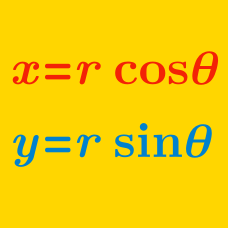Algebra

# Converting Polar Coordinates to Cartesian

The point $$\left(12, \frac{3\pi}{4} \right)$$ in polar coordinates can be expressed as $$(a, b)$$ in Cartesian coordinates, where $$a$$ and $$b$$ are real numbers. What is the value of $$-ab?$$

Suppose that $$(a, \frac{\pi}{6})$$ in polar coordinates represents the same point as $$(b, 7)$$ in Cartesian coordinates. What is the value of $$a^2+b^2$$?

$$P=(24, \frac{\pi}{3})$$ in polar coordinates can be expressed as $$P=(a, b)$$ in Cartesian coordinates. What is the value of $$a+b^2$$?

If point $$P$$ is given in polar coordinates as $$P=\left(-10, \frac{3\pi}{4}\right),$$ what are the Cartesian coordinates of $$P?$$

In polar coordinates, the graph of $$r = 46 \sin(\theta)$$ is a circle. What is the radius of this circle?

×

Problem Loading...

Note Loading...

Set Loading...